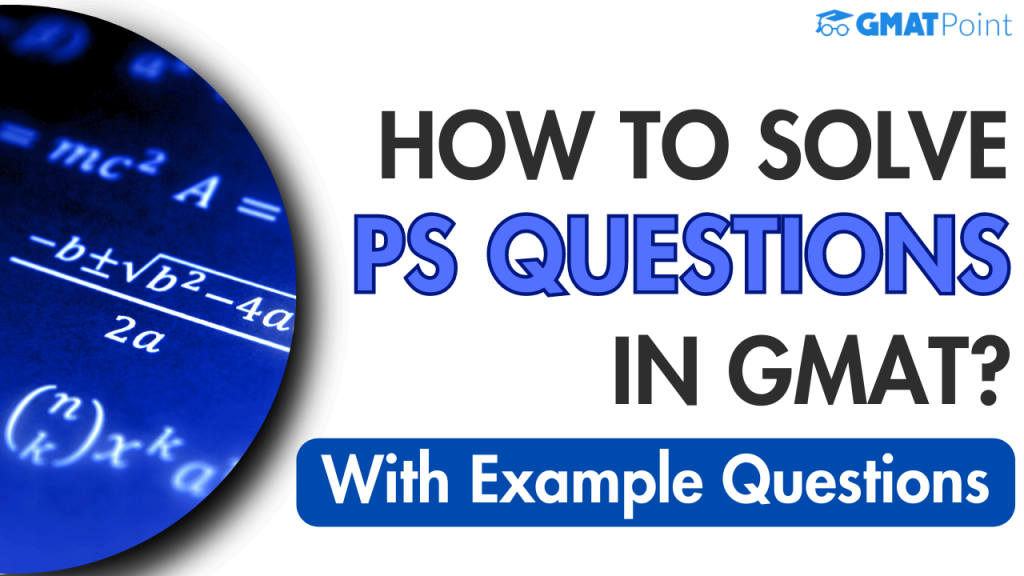# GMAT Quantitative Reasoning: How To Solve PS Questions?Out of the two most important sections in GMAT, Verbal Reasoning and Quantitative Reasoning, the latter consists of data sufficiency and problem-solving questions. Problem-solving questions require reading the problem statement and selecting the correct answer from the given options. There is no substitute for practising and disciplined learning to improve at solving these questions. A few techniques, however,  help you answer these questions more accurately. We will look at examples of solving problem-solving questions for the GMAT.

All the examples have been taken from the GMATPoint Daily Targets, which is an excellent resource for you to hone your aptitude in VR and QR. Daily targets consist of daily timed practice quizzes that are of GMAT level. After you solve the questions within the given time, you will be provided with video solutions on how to solve these questions in the best way by GMAT experts. If you are looking to take GMAT, this completely free tool will help you maintain discipline in your schedule and help you revise all the standard topics in GMAT.

Q1. Sayan bought 2 mangoes, 3 oranges, and 4 bananas for 27 rupees on Sunday. On Monday, he bought 4 mangoes, 1 orange, and 2 bananas for 31 rupees. What amount did he invest in purchasing 10 mangoes, 10 oranges, and 14 bananas?

1. 116
2. 112
3. 110
4. 120
5. 124

Answer:  We get two equations: Let the mango, orange, and banana cost M, O, and B, respectively.

It is given that the cost of 2 mangoes, 3 oranges, and 4 bananas is 27 rupees, which implies 2M+3O+4B=272M+3O+4B=27 ….. Eq(1)

Similarly, it is given that the cost of 4 mangoes, 1 orange, and 2 bananas is 31 rupees, which implies 4M+O+2B=314M+O+2B=31….. Eq(2)

We have two equations with three variables. If they are mutually inconsistent, then there cannot be a solution. However, if they are mutually consistent, then there will be exactly one solution. To verify this, we multiply Eq(1) by m and Eq(2) by n.

To find the RHS of the equation 10M+10O+14B=? we get three new equations

2m+4n=10

3m+n=10

4m+2n=14

Solving these, we get m=3, n=1

Hence the RHS of the required equation is = 27xm+31xn= 27×3+31×1=112 (Option B)

Q2. If x>0,y>0x>0,y>0, and 3x+5y=2423x+5y=242, what are the number of integer solutions of xx and yy that satisfies the equation?

1. 10
2. 12
3. 15
4. 16
5. 20

Answer: It is given that x>0,y>0x>0,y>0, and 3x+5y=2423x+5y=242. We can write this as y =   242−3x5y =  5 242−3x​

The first value of xx is 44, where the value of yy is y =   242−3⋅45=2305=46y =  5 242−3⋅4​=5230​=46.

Now, the value of xx will increase with the difference, which equals the coefficient of yy. Therefore, the next value of xx will be (4+5)=9(4+5)=9.

Similarly, the pattern of xx will be 4,9,14,19,….,794,9,14,19,….,79. Hence, when x=79x=79, the value of y is positive and integer, and when x=84, the value of y is not positive. Therefore, the last value of xx will be 7979.

Hence, the number of terms are 79=4+(n−1)5 =>(n−1)5 = 75 => n−1 =15 => n=1679=4+(n−1)5 =>(n−1)5 = 75 => n−1 =15 => n=16

Hence, the number of positive solutions is 16.

The correct option is D.

Q3. A tuition class had three batches of equal strength, with the ratio of male and female students in each batch as 3:2, 1:5, and 3:1, respectively. If a student is selected at random from this tuition class, find the probability of the student is a girl.

1. 89/180
2. 91/180
3. 83/190
4. 87/194
5. 2/3

Answer: We’re given that strength of each batch is equal, let us assume it to be 60x

In batch 1, the ratio of male and female students = 3:2

Number of female students = 2*60x/(3+2) = 24x

In batch 2, the ratio of male and female students = 1:5

Number of female students = 5*60x/(1+5) = 50x

In batch 3, the ratio of male and female students = 3:1

Number of female students = 1*60x/(1+3) = 15x

Total number of female students = 24x+50x+15x = 89x

Total number of students = 60x*3 = 180x

Hence, probability of selecting a female student = 89x/180x = 89/180 (Option A)

To solve more such challenging GMAT-level questions, log on to GMATPoint and take the free Daily Targets today.